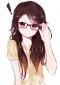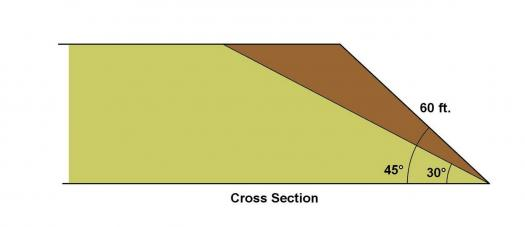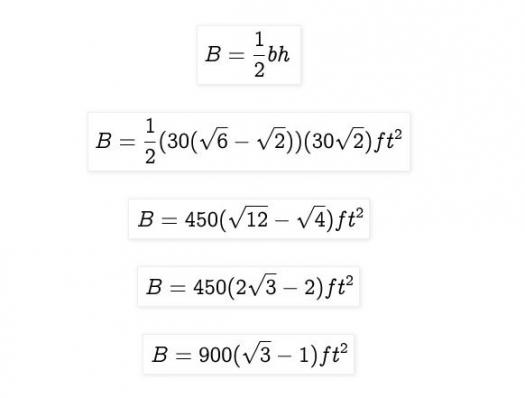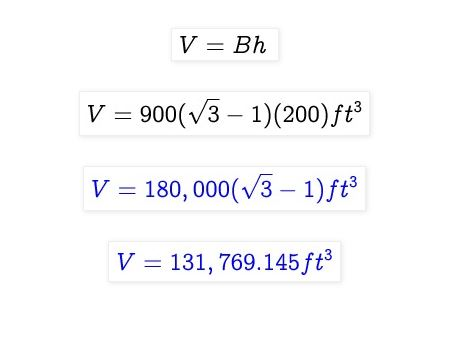# prism

2 posts / 0 new
eMATHure21prism

help me solve this problem please...

Pennsylvania Railroad found it necessary, owing to land slide upon the road bed, to reduce the angle of inclination of the bank of a certain railway cut near Pittsburgh, from an original angle of 45 to a new angle 30.the bank as it originally stood was 200 ft long and had a slant length of 60 ft. find the amount of the earth removed, if the top level of the bank remained unchanged..?
--kindly show the solution. thank you!

fitzmerl duronTo answer the problem above, we need a figure...The given figure is the cross section of a bank. The darker area of the cross section will be removed so that the inclination will be $30^o$ instead of $45^o$. The darker area is also an obtuse triangle and we need to find the area of that one. Let's start to get the sides of the triangle by analyzing the figure as followsConsider the $45^o$ triangle in order to solve for h and c as follows:Consider the $30^o$ triangle in order to solve for d as follows:The base of a triangle can be calculated as follows:The area of a triangle is also the area of the base is calculated as follows:Finally, the volume of a prism is also the amount of the soil or earth removed from the bank is calculated as follows:Credits goes to this site: Math Principles in Everyday Life

Alternate solutions are encouraged....hehehehe

Subscribe to MATHalino on